Related Articles

# Working with Date and Time in Julia

• Last Updated : 28 Jul, 2020

Julia provides a library to use Dates for dealing with date and time. The Dates module comes inbuilt with the Julia we just need to import it in order to use it. Now we need to prefix every function with an explicit type Dates, e.g Dates.Date. If you don’t want to prefix on each function just add using Dates in your code and then you will be able to use any function of Dates without using Dates as a prefix.

The Dates module provides supplies classes to work with date and time. There are many functions available that are used to manipulate Date and Time in Julia.

For working with Date the DateTime provides two main Modules: Date and DateTime are mostly used. They both are subtypes of the abstract TimeType.

#### Getting Different Types of Dates

• Dates.Date( year, month, date): It returns date with provided parameters. It is independent of time zones.
• Dates.Date(year): It returns date with the provided year, and the month, the day is set to 01
• Dates.Date(year, month): It returns date with the provided year and month, and the date is set to 01.
• Dates.DateTime( year, month, date, hour, min, sec): It returns date and time and it specifies the exact moment in time. The accuracy is to a millisecond.
• Dates.today(): It provides the Date object for the current date
• Dates.now(): It returns a DateTime object for the current instance in time.
• Dates.now(Dates.UTC): It returns date with Coordinated Universal Time (UTC) time zone.

For Example:

## Julia

 `# import Dates module``import` `Dates`` ` `# returns date with provided parameter and independent of time zone``d ``=` `Dates.Date(``2001``, ``12``, ``12``)`` ` `# returns a date with the provided year, and the month and day are set to 01``d ``=` `Dates.Date(``2001``)`` ` `# returns a date with the provided year and month, and the day is set to 01``d ``=` `Dates.Date(``2001``, ``11``)`` ` `# returns date and time and it specifies the exact moment in time. The accuracy is to a millisecond.``d ``=` `Dates.DateTime(``2001``, ``10``, ``1``, ``12``, ``11``, ``11``) `` ` `# returns the Date object for the current date``d ``=` `Dates.today()`` ` `# returns a DateTime object for the current instance in time``d ``=` `Dates.now()`` ` `# returns date with Coordinated Universal Time (UTC) time zone``d ``=` `Dates.now(Dates.UTC) `

The output of the following code: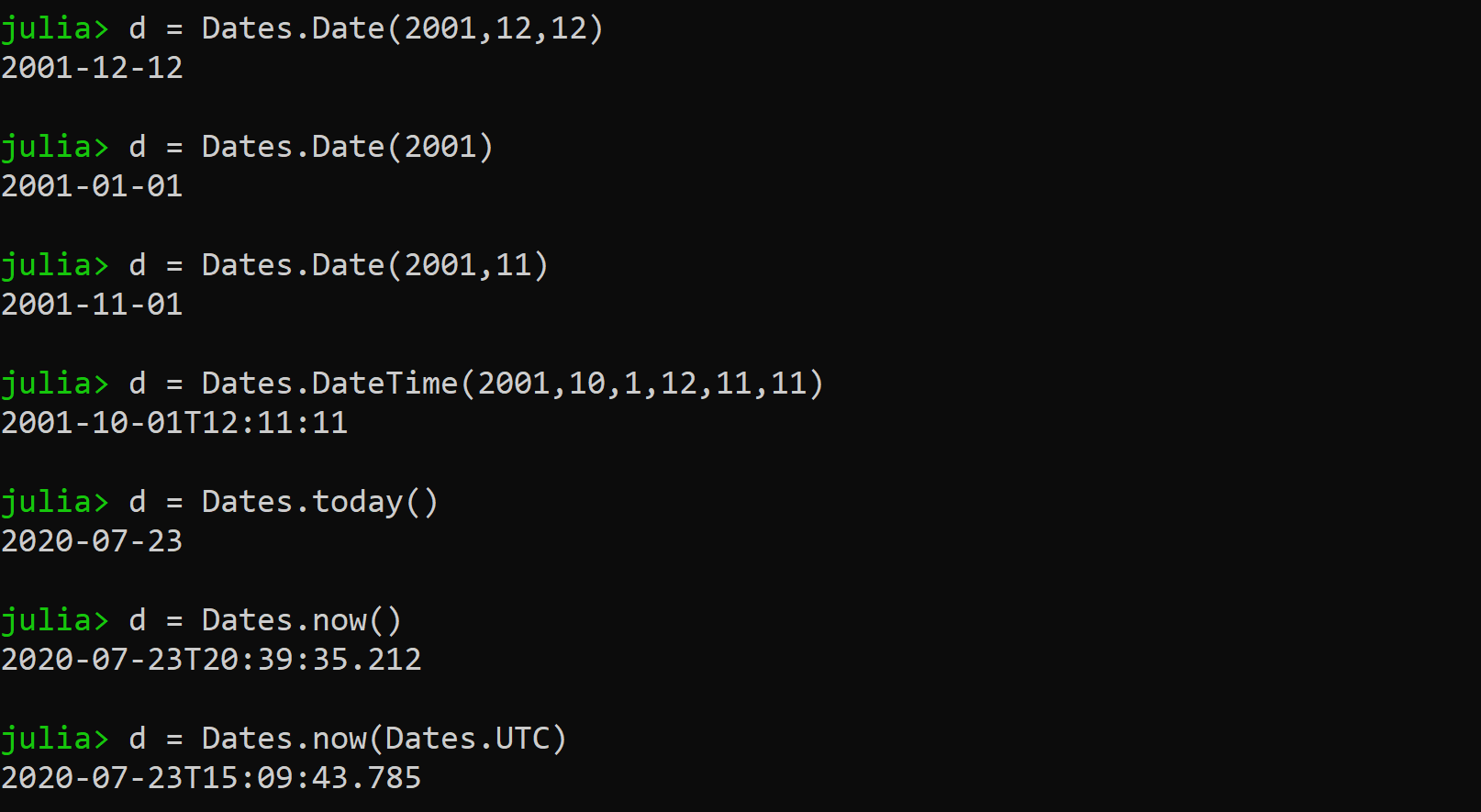Different_types_of_dates

#### Formatting Of Dates

In order to format Dates, we need to refer the characters which represent/refer date elements. Here is a table for referring to what each character represents.

We can  these formatting strings with functions such as DateTime(), Dates.format() and Date() The following examples will show various date formatting methods:

• Using DateTime()

## Julia

 `import` `Dates`` ` `# formatting a string into a DateTime() ``Dates.DateTime(``"Tue, 11 Jan 2001 12:10:4"``, ``"e, d u y H:M:S"``)`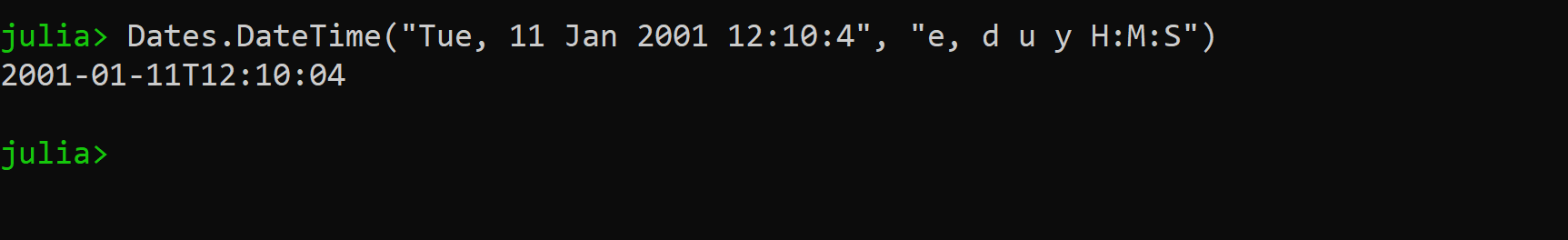DateTime example

• Using Date()

## Julia

 `import` `Dates`` ` `# Convert a string date to a d/m/yyyy format``Dates.Date(``"Mon, 12 Jan 2002"``, ``"e, d u y"``)`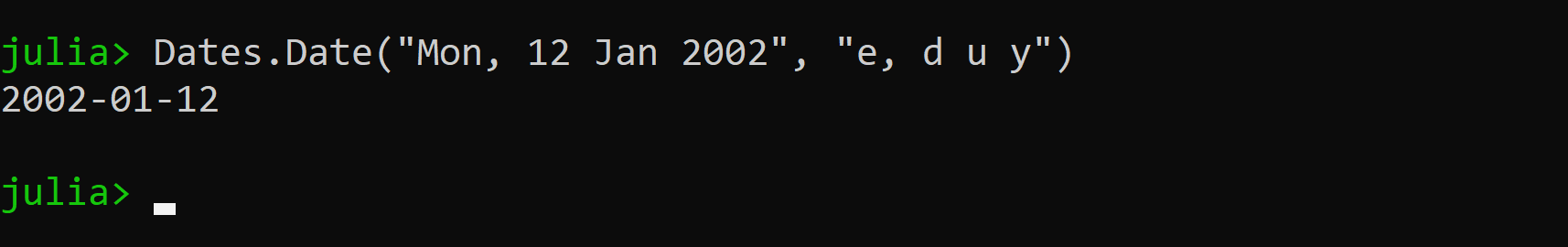Date_Example

• Using format()

## Julia

 `import` `Dates`` ` `# getting current time``my_time ``=` `Dates.now()    `` ` `# using format() to format string into time``Dates.``format``(my_time, ``"e, dd u yyyy HH:MM:SS"``)`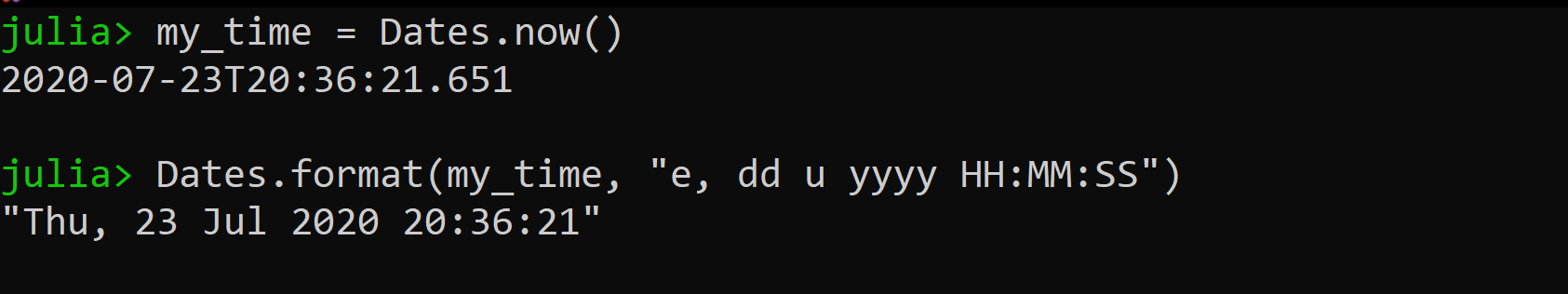format_example

• Sometimes we require leading zeros for single date element. This example shows how we can add leading zero.

## Julia

 `import` `Dates`` ` `my_time ``=` `Dates.DateTime(``"Mon, 1 Jun 2001 1:2:4"``, ``"e, d u y H:M:S"``)`` ` `# with leading zeros``Dates.``format``(my_time, ``"e: dd u yy, HH.MM.SS"``) `` ` `# without leading zero``Dates.``format``(my_time, ``"e: d u yy, H.M.S"``)`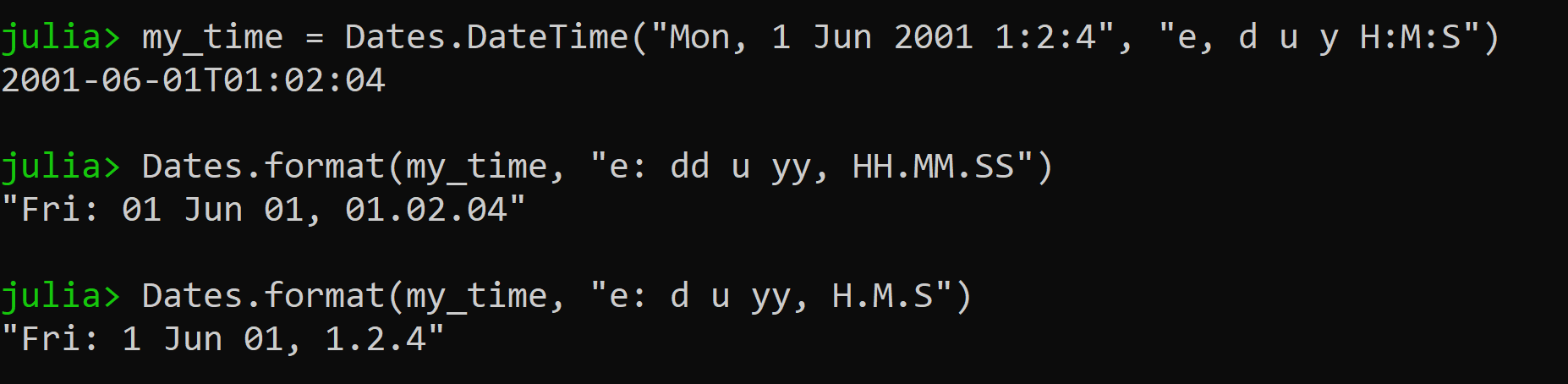• Also, we can convert a date string from one format to other by using DateTime() to convert into DateTime object and then use DateFormat() to display/output to a different format.

## Julia

 `import` `Dates`` ` `# date that needs to be formatted``date ``=` `"Mon, 12 Jul 2001 12:13:14"`` ` `# using DateTime() to obtain DateTime() obj.``temp ``=` `Dates.DateTime(date, ``"e, dd u yyyy HH:MM:SS"``)`` ` `# Using Dates.format()``Dates.``format``(temp, ``"dd, U, yyyy HH:MM, e"``)`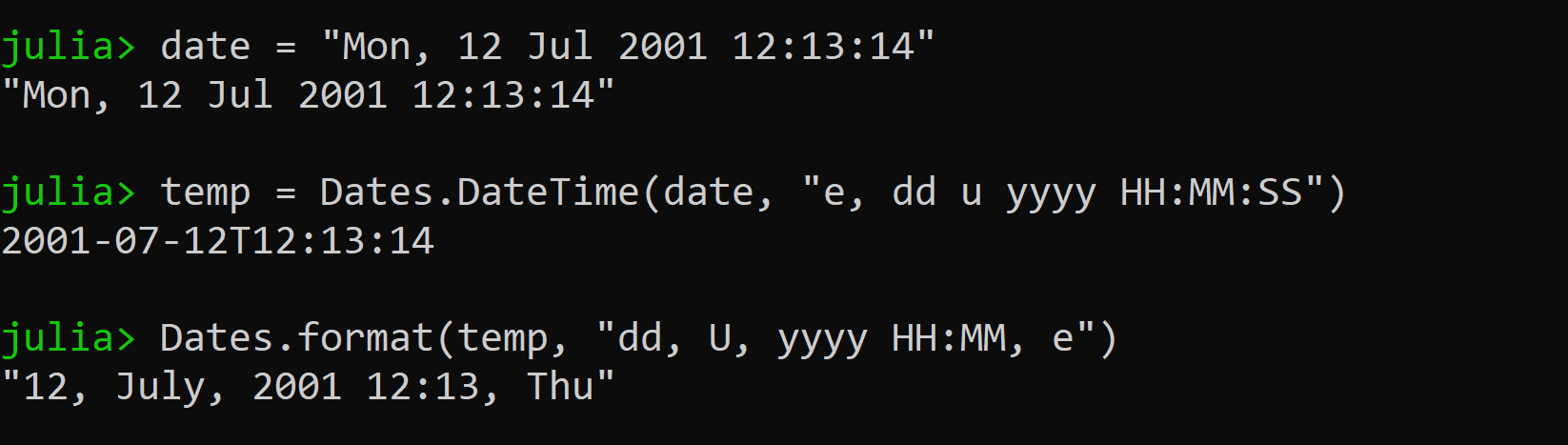one_form_to_another

• When we have a lot of dates to handle we use a DateFormat object to handle an array of string dates

## Julia

 `import` `Dates`` ` `# defining general format for dates``dateformat ``=` `Dates.DateFormat(``"y-m-d"``);`` ` `Dates.Date.([  ``      ``"2001-11-01"``, ``      ``"2002-12-12"``, ``      ``"2003-02-6"``, ``      ``"2004-04-7"``, ``      ``"2005-12-9"``, ``      ``"2006-11-12"``      ``], dateformat)`` `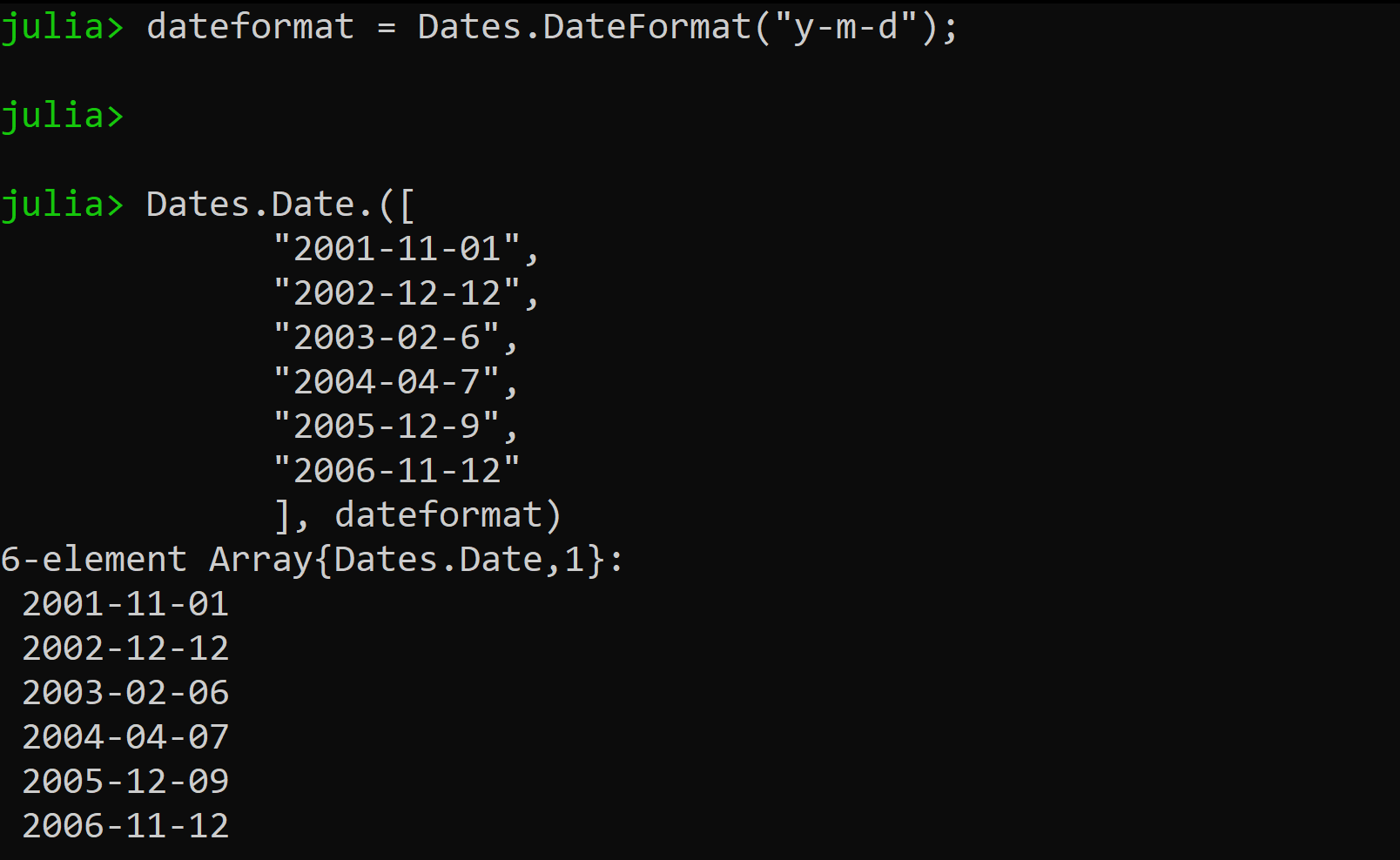array_of_dates

• We can also use Dates.ISODateTimeFormat which provides an ISO8601 format. The example is given below:

## Julia

 `import` `Dates`` ` `# ISODateTimeFormat``Dates.Date.([  ``      ``"2001-11-01"``, ``      ``"2002-12-12"``, ``      ``"2003-02-6"``, ``      ``"2004-04-7"``, ``      ``"2005-12-9"``, ``      ``"2006-11-12"``      ``], Dates.ISODateTimeFormat)`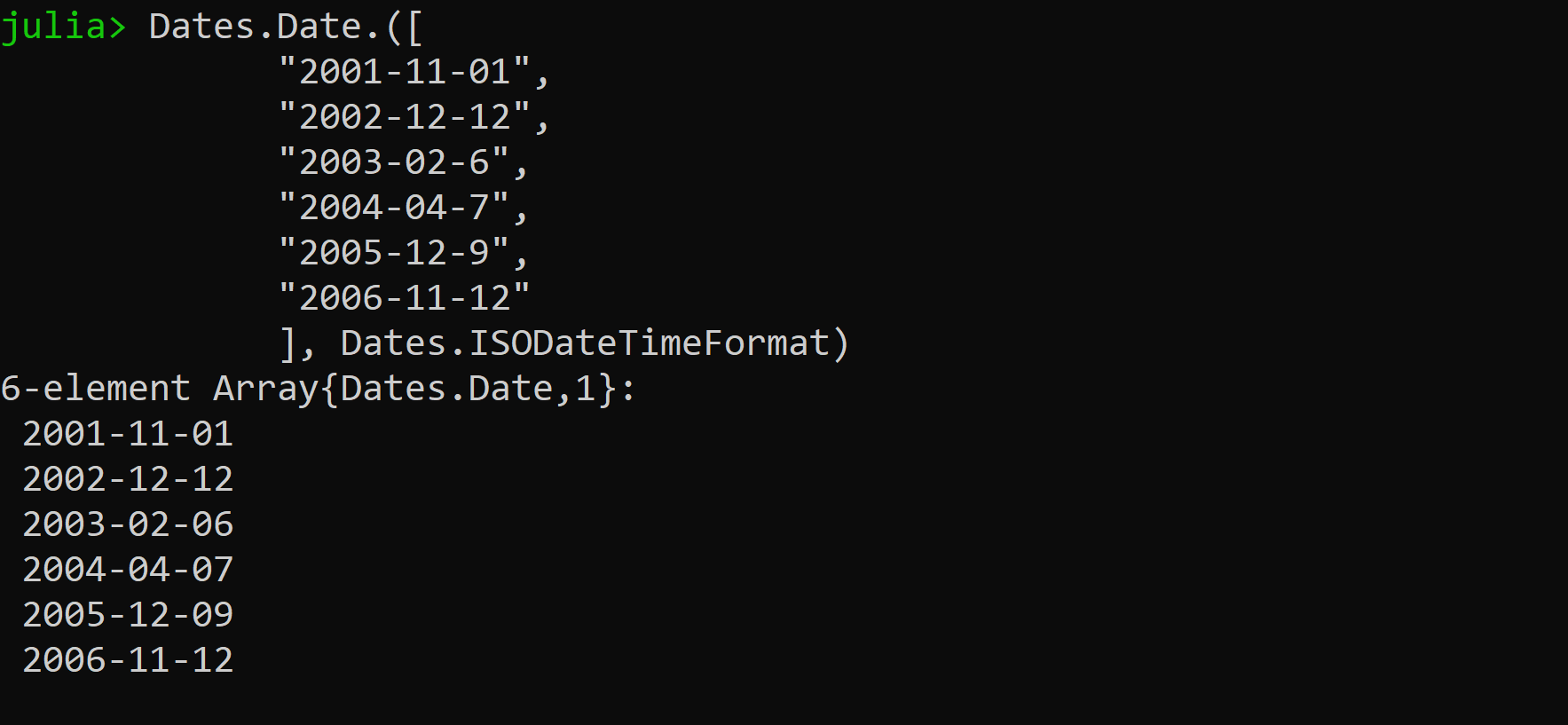ISOFORMAT_format_example

#### Getting Different Types of Time

For obtaining time in Julia we use Dates.Time() function mostly. which follows the proleptic Gregorian calendar. The different types of time are as follows:

• Dates.Time(Dates.now())  it returns a time in HH:MM:SS:ss and it takes Date object.
• Dates.minute( t) it gives the minute of the Dates.Time object passed.
• Dates.hour(t) it gives the hour of the  Dates.Time object passed.
• Dates.second(t) it gives the second of the Dates.Time object passed.

## Julia

 `import` `Dates`` ` `# creating a Dates object``t ``=` `Dates.now()`` ` `# Provides the current time``Dates.Time(t)`` ` `# Provides the hour of date object``Dates.hour(t)`` ` `# Provides the second of date object``Dates.second(t)`` ` `# Provides the minutes of date object``Dates.minute(t)`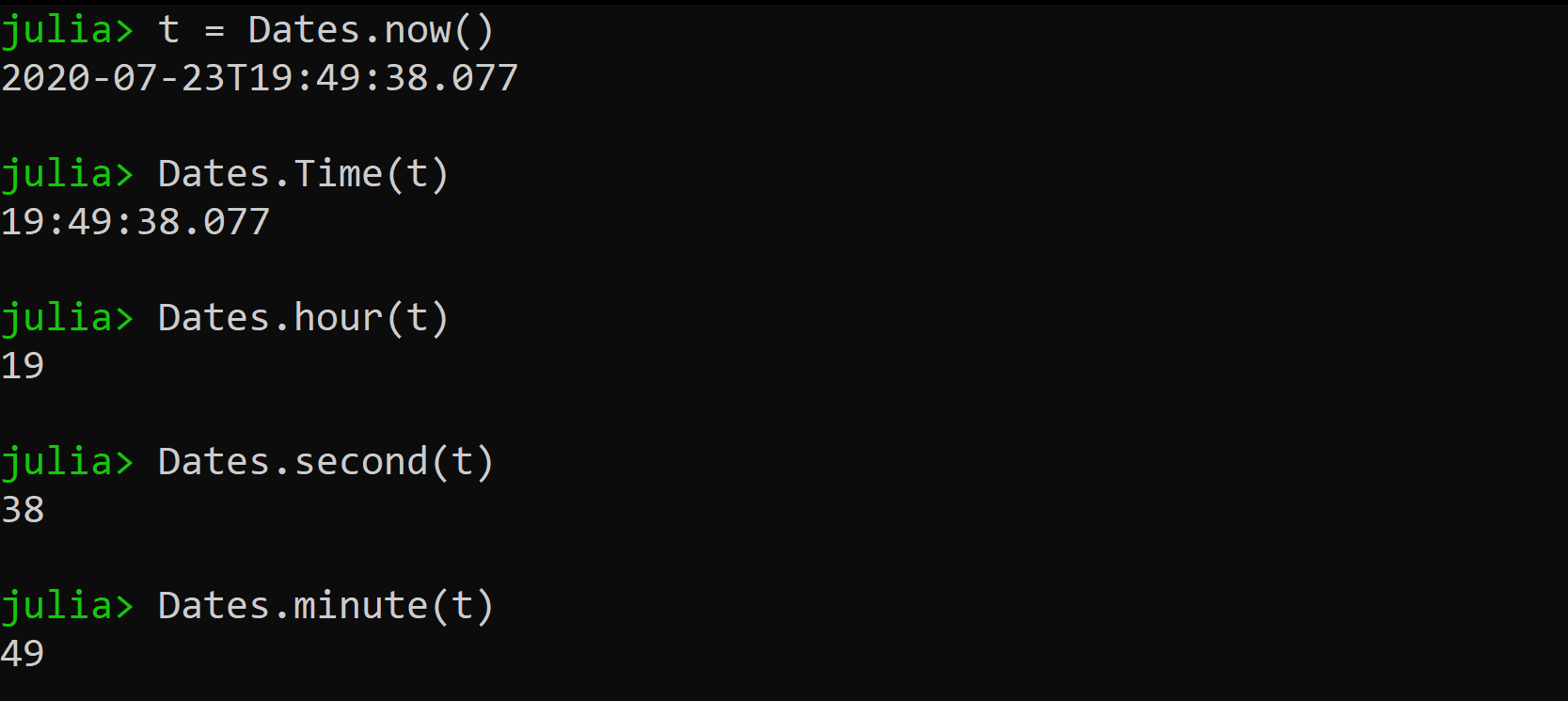time_module_example

#### Epoch Time

It is also referred to as UNIX time. It is used to deal with timekeeping. It is a count of the number of seconds that have elapsed since the beginning of the year 1970.

• The time() function, when used without any arguments, returns the Unix/Epoch time value of the current second.

## Julia

 `# provides unix time of current second``time()`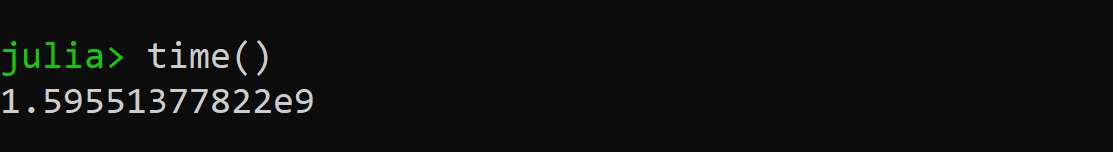time()_output

• To convert UNIX time into more readable form we use strftime() (“string format time”) function.

## Julia

 `# 1 year worth of UNIX time``Libc.strftime(``86400` `*` `365.25` `*` `1``)`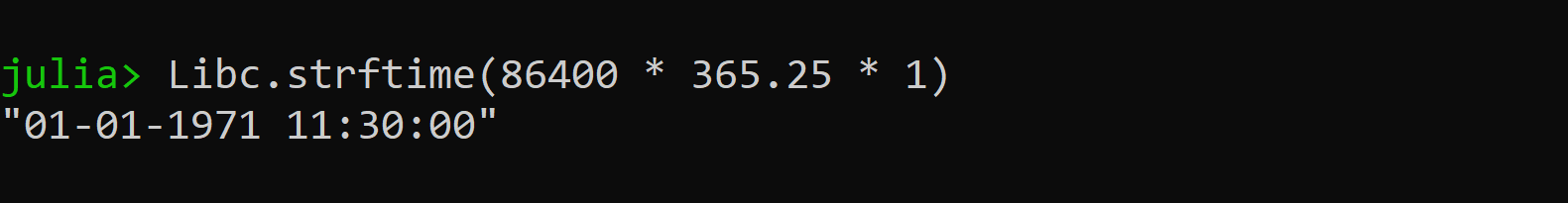strftime() example

• The function unix2datetime() converts a UNIX time into date/time object.

## Julia

 `import` `Dates`` ` `# To convert from unix to date/time object``Dates.unix2datetime(time())`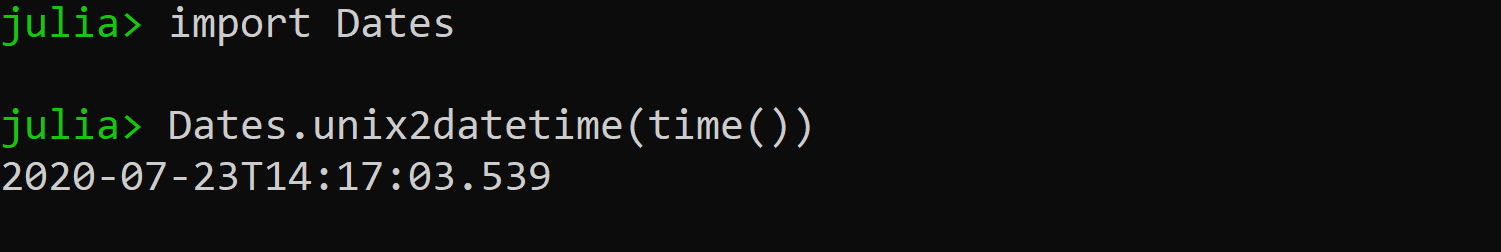unix2datetime() example

There are many other functions for manipulating date and time. Refer to the official documentation to learn more about different implementation.

My Personal Notes arrow_drop_up